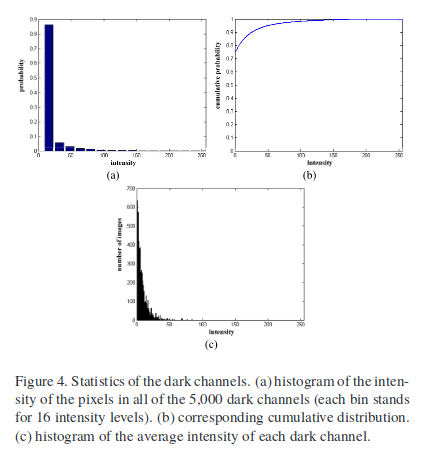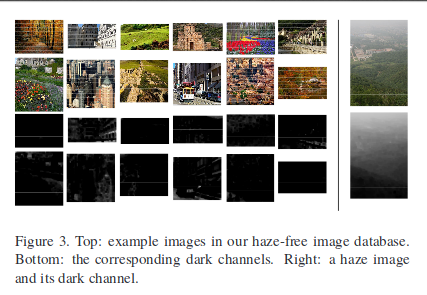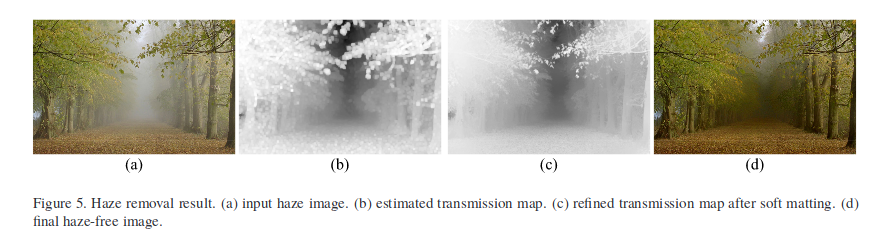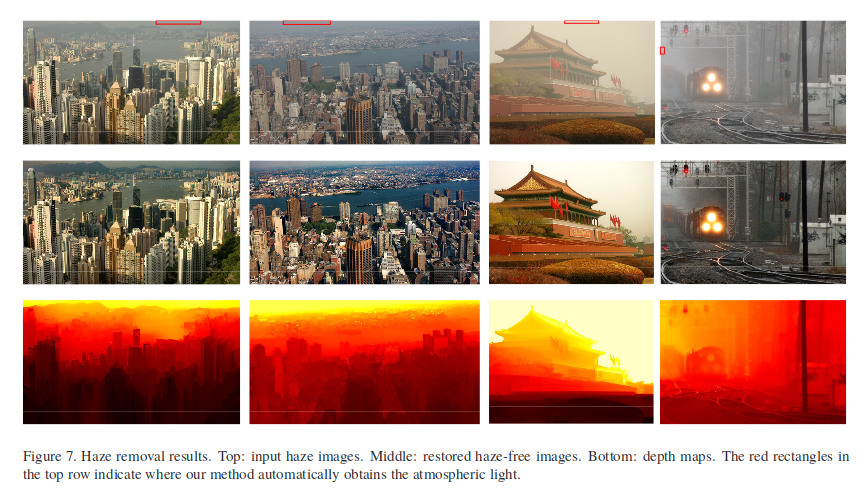### Basic Idea$$\min _{c}\left(\min _{\mathbf{y} \in \Omega(\mathbf{x})}\left(\frac{I^{c}(\mathbf{y})}{A^{c} }\right)\right)$$正是经过normalize的雾气图$$\frac{I^{c}(\mathbf{y})}{A^{c} }$$的暗通道图，因此我们可以直接得到对于透射率的估计。在这里我们可以加另外一个参数$$\omega$$，因为透射率是1的情况很少，会使得照片显得不自然而且损失一些信息，因此我们可以通过调整$$\omega$$来使得结果更加自然，最终得到的透射率估计为: $\tilde{t}(\mathbf{x})=1-\omega \min _{c}\left(\min _{\mathbf{y} \in \Omega(\mathbf{x})}\left(\frac{I^{c}(\mathbf{y})}{A^{c} }\right)\right)$ ### Soft Matting### 恢复原图